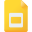# mathematics high school united states tutorialsRational Expressions, Vertical Asymptotes, and Holes
Rational Functions, High School, United States, MathematicsDerivative of a Function
Derivative, High School, United States, Differential Equations, MathematicsRules for Differentiation
Differentiation, High School, United States, MathematicsThe Mean Value Theorem
Derivative, High School, United States, MathematicsBasics of Geometry ( POINTS, LINES, PLANES! )
Geometry, High School, United States, MathematicsGeometric Shapes
Geometry, High School, United States, MathematicsCircles and Segments related to Circles
Circle, High School, United States, Lines and Angles, MathematicsProduct Rule and Quotient Rule
Differential Equations, High School, United States, Derivative, MathematicsCommon and Natural Logarithms
Logarithmic Functions, High School, United States, MathematicsLogistic Growth Model of an Exponential Function
Logarithmic Functions, High School, United States, Integral Calculus, MathematicsGreatest Common Factors: Factoring by Grouping
Factor, High School, United States, MathematicsMore About Parabolas and Their Applications
Functions and Graphs, High School, United States, MathematicsGraphing with Calculus and Calculators
Calculus, High School, United States, Mathematics, GraphingLinear Equations in Two Variables
Linear Algebra, High School, United States, MathematicsIntegration of Rational Functions by Partial Fractions
Methods of Integration, High School, United States, Integral Calculus, MathematicsRadical Expressions, High School, United States, MathematicsExponential Functions
Exponential Functions, High School, United States, MathematicsThe Rectangular Coordinate System
Mathematics, High School, United States, Functions and Graphs, Linear and Quadratic EquationsThe Fundamental Theorem of Calculus
Calculus, High School, United States, MathematicsPYTHAGOREAN THEORAM
Pythagorean Theorem, High School, United States, MathematicsThe Chain Rule
Derivative, High School, United States, Chain Rule, MathematicsIntroduction to Limits
Limits and Continuity, High School, United States, MathematicsCalculating Areas and Distances using Integrals
Integral Calculus, High School, United States, MathematicsThe Power Rule and other Rules for Differentiation
Differentiation, High School, United States, Derivative, MathematicsQuadratic Equation, High School, United States, MathematicsFinding Volumes Using Integrals
Integral Calculus, High School, United States, MathematicsTechniques for Evaluating Limits
Limits and Continuity, High School, United States, MathematicsGeometric Sequence and Series
Geometric Progression, High School, United States, MathematicsFinding Limits Analytically
Limits and Continuity, High School, United States, MathematicsIntegration by Substitution and Separable Differential Equations
Substitution Rule, High School, United States, Methods of Integration, Integral Calculus, MathematicsIndefinite Integrals and the Net Change Theorem
Indefinite Integral, High School, United States, Calculus, MathematicsImplicit Differentiation
Differentiation, High School, United States, Derivative, MathematicsTrigonometry Overview
Trigonometry, High School, United States, MathematicsSpecial Factoring
Factor, High School, United States, MathematicsModeling with Differential Equations
Differential Equations, High School, United States, Ordinary Differential Equation, MathematicsDefine Logarithmic Functions
Logarithmic Functions, High School, United States, MathematicsRatio and Proportions
Mathematics, High School, United States, Ratio and ProportionLinear Differential Equations of First Order
Differential Equations, High School, United States, Ordinary Differential Equation, MathematicsLinear Equations in Two Variables
Linear and Quadratic Equations, High School, United States, Mathematics, Linear AlgebraThe Complex Number System
Mathematics, High School, United States, Complex NumbersDerivatives of Logarithmic Functions
Derivative, High School, United States, Mathematics, Logarithmic FunctionsLimits and Continuity
Limits and Continuity, High School, United States, MathematicsComplex Numbers
Mathematics, High School, United States, Complex NumbersThe Substitution Rule in Integration
Integral Calculus, High School, United States, Mathematics, Substitution Rule, Methods of IntegrationCurve Sketching using Calculus
Applications of Derivatives, High School, United States, Mathematics, Calculus, Curve SketchingRational Expressions and Functions; Multiplying and Dividing
Rational Functions, High School, United States, MathematicsFunctions and their Graphs
Mathematics, High School, United States, Functions and GraphsProperties of Logarithms
Logarithmic Functions, High School, United States, MathematicsIntegration By Parts
Integral Calculus, High School, United States, Methods of Integration, MathematicsRates of Change and Limits
Mathematics, High School, United States, Limits and ContinuityMathematics, High School, United States, Radical ExpressionsOperation on Real Numbers
Mathematics, High School, United States, Real NumbersEquations with Rational Expressions and Graphs
Rational Functions, High School, United States, MathematicsExponential and Logarithmic Equations; Further Applications
Logarithmic Functions, High School, United States, MathematicsArithmetic Sequence and Series
Arithmetic, High School, United States, MathematicsFactoring Trinomials
Factor, High School, United States, MathematicsAreas Between Curves using Definite Integrals
Definite Integral, High School, United States, Calculus, Mathematics, Applications of IntegralsHow to Calculate Average Value of a Function using Definite Integrals
Integral Calculus, High School, United States, Applications of Integrals, MathematicsPolynomial Functions
Polynomial Functions, High School, United States, MathematicsWhat is CALCULUS? - An Introduction
Calculus, High School, United States, MathematicsEquations and Inequalities
Mathematics, High School, United States, InequalityMultiplying the Polynomials
Mathematics, High School, United States, Polynomial FunctionsInverse Functions
Polynomial Functions, High School, United States, MathematicsCalculate Volume of Cylindrical Shells using Integration
Applications of Integrals, High School, United States, Integral Calculus, Definite Integral, MathematicsLimits for Functions of One and Two Variables
Polynomial Functions, High School, United States, Limits and Continuity, MathematicsStrategies for Integration
Methods of Integration, High School, United States, Integral Calculus, MathematicsComplex Fractions: Objectives and Examples
Fractions and Multiplication, High School, United States, Mathematics, AlgebraEquations of a Circle
Mathematics, High School, United States, Equation of CircleDirection Fields and Euler’s Method
Differential Equations, High School, United States, Ordinary Differential Equation, MathematicsIntegration by Trigonometric Substitution
Integral Calculus, High School, United States, Mathematics